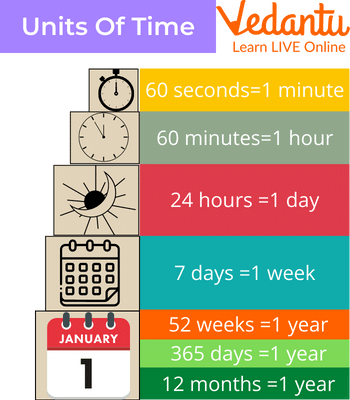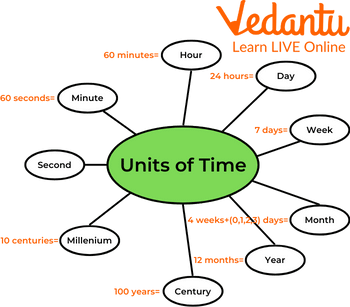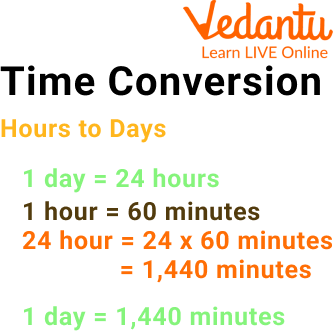Courses
Courses for Kids
Free study material
Free LIVE classes
More

# Units of TimeLIVE
Join Vedantu’s FREE Mastercalss

## An Introduction to the Units of Time

Everywhere in the world, time is measured using the same system. What exactly is that? How many seconds in a day? What is the SI unit of time? Well, you can see for yourself if you simply look at a watch or a clock. Do you see anything? Minutes and hours are displayed. What else do you notice? Also visible are seconds.

Since they are used to measure time, they are referred to as units of time. The seconds hand travels the fastest, followed by the minute and hour arms if you take some time to observe an extremely old clock with moving arms. Your clock will only display 60 seconds and 60 minutes, as you will also observe.Units of Time

For hours, just 12 are displayed. When converting between your time units of hours, minutes, and seconds, you must take into account how different the time system operates.

## Knowing the Units of TimeUnits of Time

The more typical time units are first displayed in the form of a concept web, going from the smallest unit to the largest unit. Start with a second, then move on to a minute, and so on.

The fundamental units of time that we are familiar with are the second, minute, hour, day, week, month, year, century, and millennium. The concept web's second unit is the smallest. We mostly use seconds, minutes, hours, days, weeks, months, and years to measure time:

 1000 milliseconds = 1 second 60 seconds = 1 minute 60 minutes = 1 hour 24 hours = 1 day 7 days = 1 week 28, 29, 30 or 31 days = 1 month 365 or 366 days = 1 year 12 months = 1 year 10 years = 1 decade 100 years = 1 century 1000 years = 1 millennium

## SI Unit of TimeMeasuring Instruments

The second, which is often known as the SI unit of time, is more precisely described as "the time interval equal to 9192631770 periods of the radiation corresponding to the transition between the two hyperfine levels of the ground state of the cesium-133 atom". Whatever the case, the unit second is sometimes written as s or sec.

## Smallest Unit of TimeSmallest Unit of Time

The smallest unit of time is the second. The base unit of time in the SI unit is second. The following can be used to express all other time units in terms of seconds:

1 minute = 60 s

1 hour = 3600 s and so on.

So, how many seconds in a day?

There are 60 seconds in a minute, 60 minutes in an hour, and 24 hours in a day. There are 86,400 seconds in a day because $60 \times 60 \times 24$.

## Minutes and Hours to Days ConversionHours and Minutes to Days Conversion

Divide the time by the conversion ratio to convert a measurement of an hour to a measurement of a day.

Since 24 hours make up one day, you can convert using the following easy formula:

days = hours ÷ 24

Hours are converted to days by dividing by 24.

For illustration, use the formula above to convert 5 hours to days.

5 hr = (5 ÷ 24) = 0.208333 days

If we want to convert minutes in a day then again we have to divide by 60.

A few units used to measure time are hours, minutes and days.

### Solved Example

1. Convert the following:

i) 7 days 6 hours into hours.

Ans: 1 day = 24 hours

$\text { Therefore, } 7 \text { days } 6 \text { hours } =(7 \times 24) \text { hours }+6 \text { hours }$

$=168 \text { hours }+6 \text { hours }$

$=174 \text { hours }$

ii) 5 years 9 months into months.

Ans: 1 year =12 months

$\text { Therefore, } 5 \text { years } 9 \text { months } =(5 \times 12) \text { months }+9 \text { months }$

$=(60+9) \text { months }=69 \text { months }$

### Practice Problem

1. Convert the following:

i) 6 hours 40 minutes into minutes.

Ans: 400 minutes

ii) 4 minutes 25 seconds into seconds.

Ans: 265 seconds

## Summary

The duration of events or the gaps between them can be measured, compared, or even ordered using time. Units must be specified to measure time; however, there are many possible time units, some of which may be more suitable than others depending on the situation.

Outside of strictly scientific purposes, other units are usually applied for longer durations.

• Minute (60 seconds)

• Hour (60 minutes, or 3,600 seconds)

• Day (24 hours, or 86,400 seconds)

• Week (7 days, or 604,800 seconds)

• Month (28-31 days, or 2,419,200-2,678.400 seconds)

Last updated date: 20th Sep 2023
Total views: 94.5k
Views today: 1.94k

## FAQs on Units of Time

1. What does a unit of time look like and what is the SI unit of time?

The following are some of the most typical and often used units: second, minute, hour, day, week, month, and year. When extended periods are involved, multiple years are also employed to designate a certain period.

The unit of time measurement used by the International System of Units (SI) is the second (s or sec). The radiation produced by the change between two levels of the cesium-133 atom undergoes 9,192,631,770 (or 9.192631770 x 109 in decimal form) cycles in one second.

2. Is it a light-year unit of time and how are units of time converted?

A light-year measures distance rather than time (as the name might imply). A light-year is the distance a light beam travels in one year on Earth, which is roughly 6 trillion miles (9.7 trillion kilometres).

Time Unit Conversion-

1 Minute = 60 seconds.

1 Hour = 60 minutes.

1 Day = 24 hours.

1 Week = seven days.

12 Months = one year.

1 Year = 52 weeks.

1 Year = 365 days (366 for leap years)

3. State some facts about time.

The following are some facts about time:

• One can use a watch, clock, hourglass, or even a sundial to measure the passing of time.

• A sundial is a device that calculates time-based on the location of the Sun and usually involves a shadow cast across a designated surface.

• A decade is defined as 10 years, a century as 100 years, and a millennium as 1000 years.

• Microseconds, nanoseconds, and milliseconds are a few examples of very little intervals of time.

• There are different time zones spread over the globe. This means that someone eating dinner elsewhere  in the world is doing so when you are eating breakfast.